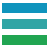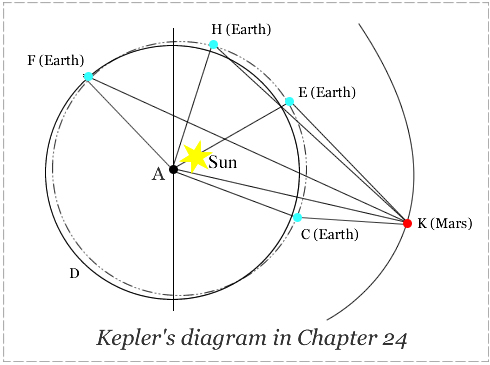Earth's MotionKepler next examined the motion of the Earth using Brahe's observations of Mars. He postulated that the Earth does not move around the center of its orbit at a uniform speed. “This is in a way surprising,” writes Donahue, “because ever since the time of Hipparchus” (second century BCE) a theory of this kind, either of an Earth or Sun moving at uniform speed depending on which was at the center, “had been used with no problems.”

Even Tycho Brahe himself had approved of it on the basis of his own data.

How did Kepler determine the Earth's orbit, using observations of Mars?

Kepler knew that the time of Mars' orbit was 687 days. Therefore, Mars will be in the same place every 687 days. If he could find multiple observations of Mars separated by this interval of time, he could observe how the angle between Earth and Mars changed and thus learn something about the parameters of Earth's orbit.Using trigonometry Kepler discovered the relative distances between Earth, Mars, and the center of Earth’s orbit, taking the Earth-Mars distance as a constant.In Chapter 24 of the New Astronomy, Kepler reasoned as follows: Assuming that the Earth moves uniformly, all the angles around the orbit's center, "A," (see below) would be equal (FAH, HAE, EAC), since they are equally spaced in time (687 days). The distances from the Earth to the center of its supposedly circular orbit should also all be the same.

Based on Brahe’s observations Kepler calculated that angle AKE is 42°21’, and angle AEK is equal to 96°22’. Setting AK, the distance from the center of Earth’s orbit to Mars equal to a constant of 100,000 units, he determined that the relative distance AD from the Earth to the assumed center its orbit would be 67,794 units.

He computed the other distance ratios to be:
AB = 66,774
AC = 67,467
AE = 67,478

His calculations demonstrated a difference among the lengths. This indicated either that A is not the center of Earth’s circular orbit, or that the orbit is not a circle. Kepler knew the radii of a circle should all be equal.

Kepler extrapolated on the method he used in Chapter 24 to obtain critical measurements. He repeated the method of "observing the Earth from Mars" with Mars at different locations and employed more complex procedures to obtain distance measures from Mars to the Sun in Chapters 26, 27, and 28 of the New Astronomy. The measures obtained in this manner later allowed him to investigate the shape of Mars' orbit.

As a result of his geometrical investigation in Chapter 24, Kepler concluded: “Therefore, the circle [D], which Copernicus described about the point [A] of uniformity of the Earth’s motion, is not the Earth’s path. There is instead some other circle ... on which the Earth is found.” (Donahue translation, p. 319)